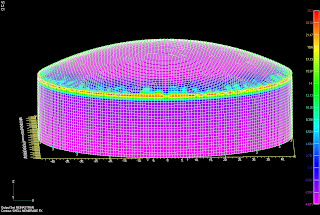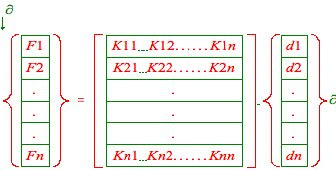An Introduction to Finite Element Analysis - An Engineer's Aspect

## Thursday, June 11, 2009

AN INTRODUCTION TO FINITE ELEMENT ANALYSIS
by Nanette South ClarkDaryl L. Logan in, “A First Course in the Finite Element Method,” Third Edition, Brooks/Cole 2002, describes the Finite Element Method as “a numerical method for solving problems of engineering and mathematical physics.” Some typical problems that are solvable by use of Finite Element methods include structural analysis, heat transfer, fluid flow, mass transport, and electromagnetic potential.

It is very difficult to obtain closed-form solutions for complicated geometries, loadings, and material properties. Analytical solutions generally require the solution of ordinary or partial differential equations, which are not usually obtainable for complex problems. Hence, numerical methods are relied upon, such as the Finite Element method, for acceptable solutions. Numerical methods yield approximate values of the unknowns at discrete points. The process of modeling a body by dividing it into an equivalent system of smaller units (finite elements) interconnected at points common to two or more elements (nodal points or nodes) and/or boundary lines and/or surfaces is called discretization. In the finite element method, equations are formulated for each finite element and then combined to obtain the solution of the entire model.

For structural problems, the solution generally refers to determining the displacements and stresses at each node and within each element that makes up the structure when it is subjected to applied loads. When analyzing nonstructural problems, the unknown nodal values may be temperatures or fluid pressures, etc.

The general steps of the finite element method for the structural stress-analysis problem are described in Logan’s text as follows.

Step 1: Discretize and Select the Element Types.

Dividing the body into an equivalent system of finite elements with associated nodes and choosing the most appropriate element type to model most closely the actual physical behavior. The elements must be small enough to give usable results and large enough to reduce computational effort. Thus, some engineering judgment must be used. Small elements are generally desirable where the results are changing rapidly, such as where changes in geometry occur; large elements can be used where results are relatively constant.

Element choice depends on the physical makeup of the body under actual loading conditions. Judgment must be used in choosing one-, two-, or three-dimensional idealizations. Elements that are commonly employed are shown in Figure 1.

Figure 1—Various Types of Finite Elements from “A First Course in the Finite Element Method,” Third Edition, Brooks/Cole 2002:The primary line elements (Figure 1a) consist of bar (or truss) and beam elements. They have a cross-sectional area but are usually line elements. The simplest line element, called a linear link or spar element, has two nodes, one at each end. Higher order elements having three nodes or more, called quadratic, cubic, etc., also exist.The basic two-dimensional, plane, elements (Figure 1b) are loaded by forces in their own plane. They are triangular or quadrilateral elements. The simplest two-dimensional plate elements have corner nodes with straight sides or boundaries, called simplex elements. There are also serendipity higher-order, quadratic, elements with midside nodes and curved sides. These elements can have variable or constant thicknesses.The most common three-dimensional elements (Figure 1c) are tetrahedral and hexahedral, brick, elements. They are used for three-dimensional stress analysis. The basic three-dimensional elements have corner nodes only and straight sides. Higher-order elements, Lagrange elements, have midedge nodes (and possible midface nodes) and have curved surfaces for their faces.The axisymmetric element (Figure 1d) is developed by rotating a triangle or quadrilateral through 360° about a fixed axis located in the plane of the element. This element can be used when the geometry and loading are axisymmetric.

Step 2: Select a Displacement Function.

The displacement function is defined within the element using the nodal values of the element. Frequently used functions are linear, quadratic, and cubic polynomials because they are simple to work with in finite element formulation. Trigonometric series can also be used. The displacement function of a two-dimensional element is a function of the coordinates in its plane. The functions are expressed in terms of the nodal unknowns. The same general displacement function can be used repeatedly for each element.

Step 3: Define the Strain/Displacement and Stress/Strain Relationships.

Strain/displacement and stress/strain relationships are necessary for deriving the equations for each finite element. For example, in the case of one-dimensional deformation, say, in the x direction, strain εx is related to the displacement u by εx = du/dx for small strains. Stresses must be related to strains through the stress/strain relation called Hooke’s Law. Being able to define the material’s behavior accurately is most important in obtaining acceptable results. Hooke’s law, σx = Eεx, is used in stress analysis, where σx = stress in the x direction and E = modulus of elasticity.

Step 4: Derive the Element Stiffness Matrix and Equations.

1.Direct Equilibrium Method: The stiffness and element equations relating nodal forces to nodal displacements are obtained using force equilibrium conditions for a basic element, along with force/deformation relationships. This method is most easily adaptable to line or one-dimensional elements.

2.Work or Energy Methods: This method is easier for two- and three- dimensional elements. The principle of virtual work (using virtual displacements), the principle of minimum potential energy, and Castigliano’s theorem are methods frequently used.

3.Methods of Weighted Residuals: These methods are useful for developing the element equations; particularly popular is Galerkin’s method.

Any of the above methods will produce the equations to describe the behavior of an element. These equations written in compact matrix form are:

{f} = [k]{d}

where {f} is the vector of elemental nodal forces, [k] is the element stiffness matrix, and {d} is the vector of unknown element nodal degrees of freedom or generalized displacements, n. These may include actual displacements, slopes, or even curvatures.

Step 5: Assemble the Element Equations to Obtain the Global or Total Equations and Introduce Boundary Conditions.

The individual element equations generated in step 4 can now be added together using a method of superposition (called the direct stiffness method)—whose basis is nodal force equilibrium—to obtain the global equations for the whole structure. There must be consistency in the deformation of the structure, that is, elements sharing a node will have the same displacements at that node.

The final assembled or global equation written in matrix form is

{F} = [K]{d}

where {F} is the vector of global nodal forces, [K] is the structure global or total stiffness matrix, and {d} is the vector of known and unknown structure nodal degrees of freedom or generalized displacements. The global stiffness matrix [K] is a singular matrix. Thus, certain boundary conditions must be invoked (from constraints or supports) so that the structure remains in place. The applied loads have been accounted for in the global force matrix {F}.

Step 6: Solve for the Unknown Degrees of Freedom (or Generalized Displacements).

The equation {F} = [K]{d} is a set of simultaneous algebraic equations that can be written in expanded matrix form aswhere n is the total number of unknown nodal degrees of freedom.

These equations can be solved for the d’s by using an elimination method such as Gauss’s Method. The d’s are called the primary unknowns, because they are the first quantities to be determined in using the finite element method.

Step 7: Solve for the Element Strains and Stresses.

Important secondary quantities of strain and stress (or moment and shear force) can be obtained because they can be directly expressed in terms of the displacements determined in step 6. The equations given in step 3 can be used.

Step 8: Interpret the Results.

The final goal is to interpret and analyze the results for use in the design/ analysis process. The determination of locations in the structure where large deformations and stresses occur is important in making design/analysis decisions.

These steps, as described in Logan’s text, are the basis for the finite element modeling software available.